# Positive-definite function on a group

(diff) ← Older revision | Latest revision (diff) | Newer revision → (diff)

A continuous function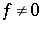on the group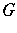such that for allinand,Examples can be obtained as follows. Letbe a unitary representation ofin a Hilbert space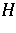, and let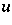be a unit (length) vector. Then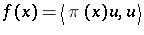is a positive-definite function.

Essentially, these are the only examples. Indeed, there is a bijection between positive-definite functions onand isomorphism classes of triples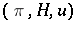consisting of a unitary representationofonand a unit vectorthat topologically generatesunder(a cyclic vector). This is the (generalized) Bochner–Herglotz theorem.

See also Fourier–Stieltjes transform (when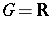).

How to Cite This Entry:
Positive-definite function on a group. Encyclopedia of Mathematics. URL: http://encyclopediaofmath.org/index.php?title=Positive-definite_function_on_a_group&oldid=18615
This article was adapted from an original article by M. Hazewinkel (originator), which appeared in Encyclopedia of Mathematics - ISBN 1402006098. See original article